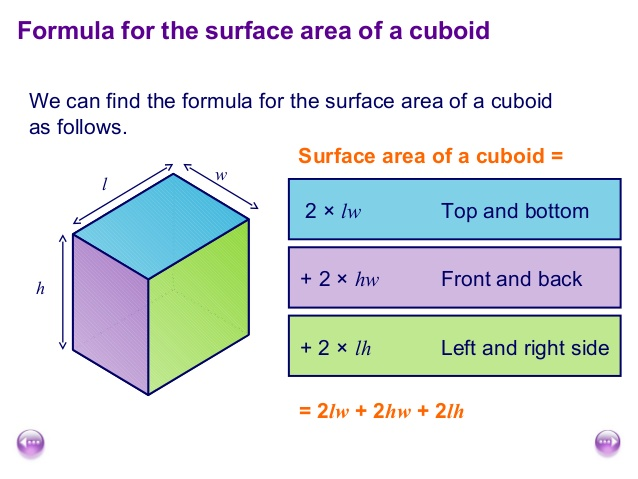# Java Program To Total Surface Area Of Cuboid | Programs

Before we show you example Java program to calculate a total surface area of a cuboid , you can check out the math formula and definition about an entire surface area of a cuboid. In case if you know the basics then skip this tutorial and move on to the program code.

Def : Total surface area of the cuboid ?

• The total surface area of a cuboid is the sum of areas of its six faces.

What is the formula to find the total surface area?

• Here goes the complete explanation:Did you get an idea, right ? Here is the program by using above method.

Program # -1 using formula ( To calculate total surface area of cuboid )

Output : ( Online execution tool soon updated )

Program # – 2 ( using command line arguments )

Here is the complete guide : Here is the complete guide on command line arguments – check here

Sample Program # 3 ( Using return Invoked method )

Java Program – Here is the complete guide

Output :

# Java program to calculate total surface area of cuboid #